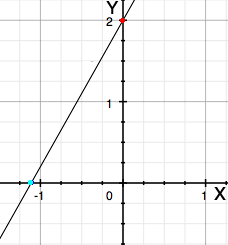SEARCH HOMEMath Central Quandaries & QueriesQuestion from connor, a student: hey im not sure how to find the x intercept in questions like 2x+3y-12=0 5x-2y-12=0 if you could show me how to find the x and the y intercepts out of this equation that would be great thanks connorHi Connor,

The intercepts identify the places where the graph crosses the axes. The x intercept identifies where the graph crosses the x-axis and the y intercept identifies where the graph crosses the y-axis. Lets look at

9x - 5y + 10 = 0.

I sketched the graph and indicated the x intercept with a blue dot and the y intercept with a red dot.I am going to find the y intercept first. Do do this I want the coordinates of the point where the line crosses the y-axis. If a point is on the y-axis then its x-coordinate is zero so set x = 0 in the equation and solve for y.

9×0 - 5y + 10 = 0
- 5y + 10 = 0
y = 2.

Hence the point where the line crosses the y-axis is (x, 2). The y intercept is the y-coordinate of this point so

the y intercept is 2.

Now that we have done it once we can find the x intercept much quicker. To find the x intercept set y = 0 and solve for x

9x - 5×0 + 10 = 0
x = -10/9.

and hence

the x intercept is -10/9.

Now try the two equations in your question to us and write back if you need more assistance,
PennyMath Central is supported by the University of Regina and The Pacific Institute for the Mathematical Sciences.